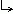Math Central - mathcentral.uregina.caProblem of the Month 2012-2013Currentproblem Recent problemswith solutions
 Older problems from 2000 to 2005 2005/2006 06/07 07/08 08/09 09/10 10/11 11/12

 PM122: April 2013Find all pairs $c$ and $d$ of real numbers such that all roots of the polynomials $$6x^2-24x-4c \quad \mbox{ and } \quad x^3 +cx^2 +dx - 8$$ are nonnegative real numbers.Solution. PM121: March 2013A unit fraction is the reciprocal $\frac1n$ of a positive integer $n$. The unit fraction $\frac1{10}$ can be represented as a difference of unit fractions in the following four ways: $$\frac1{10}=\frac15-\frac1{10}; \quad \frac1{10}=\frac16-\frac1{15}; \quad \frac1{10}=\frac18-\frac1{40}; \quad \frac1{10}=\frac19-\frac1{90}.$$ In how many ways can the fraction $\frac1{2013}$ be expressed in the form $$\frac1{2013} = \frac1x - \frac1y ,$$ where $x$ and $y$ are positive integers?Solution. PM120: February 2013Find all real-valued functions $f(x)$ such that $$f\left(x^3+y^3\right) = x^2f(x) + yf(y^2)$$ for all real numbers $x$ and $y$.Solution. PM119: January 2013You have five line segments such that every choice of three of them can be used as the sides of a triangle. (This means that for any three of the segments, the sum of the lengths of the smaller two segments must exceed the length of the third.) Prove that at least one of these ten possible triangles must be acute.Solution. PM118: December 2012Find all positive integers $n$ such that $n+184$ and $n-285$ are both cubes of integers.Solution. PM117: November 2012Let $w$ be any $n$-letter "word", where by word we just mean a collection of letters written together — it does not have to be a word in any known language. Prove that if $w$ contains at most 10 different letters, such as monthlyproblm, then you can replace the letters of $w$ by decimal digits (different letters replaced by different digits, same letters by same digit) so that the resulting $n$-digit number is a multiple of 3. For example, if we let $m=1, \; o = 2$, and so on, we get the number 1234567892061, which is a multiple of 3. Give an example of a word containing at most 10 different letters that is not a multiple of 7 by any assignment of the 10 decimal digits to its letters (again, different letters replaced by different digits, same letters by same digit)Solution. PM116: October 2012We say that a real-valued function $f(x)$ of the real variable $x$ is strictly decreasing if $f(a) > f(b)$ whenever $a < b$. Does there exist a strictly decreasing function $f$ for which $f\left(f(x)\right) = x+1$ for all $x\in \mathbf{ R}$? Does there exist a strictly decreasing function $g$ for which $g\left(g(x)\right) = 2x+1$ for all $x\in \mathbf{ R}$?Solution. PM115: September 2012$N$ is a set containing some of the counting numbers from 1 to 15; it has the property that the product of any three of its numbers is not a square. For example, if $N$ were to contain 2 and 6, then it could not contain 12 because $2\cdot 6 \cdot 12 = 144$, which is a square. How large can $N$ possibly be?Solution.
 Math Central is supported by the University of Regina and the Imperial Oil Foundation.about math central :: site map :: links :: notre site français* Registered trade mark of Imperial Oil Limited. Used under license.